##### Question

In: Statistics and Probability

# Many elementary school students in a school district currently have ear infections. A random sample of...

Many elementary school students in a school district currently have ear infections. A random sample of children in two different schools found that 21 of 42 at one school and 15 of 35 at the other had this infection. Conduct a test to answer if there is sufficient evidence to conclude that a difference exists between the proportion of students who have ear infections at one school and the other. Find the test statistic.

## Solutions

##### Expert Solution

Let P1 be the proportion of students who have ear infections at one school,

and P2 be the proportion of students who have ear infections at other school.

Hypothesis :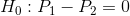v/s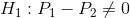Given data :

X1=21

n1=42

X2=15

n2=35

Sample proprotions are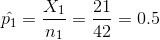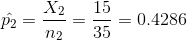Pooled proportions: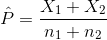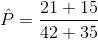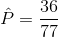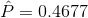Test statistics :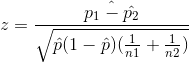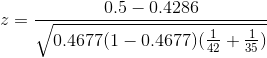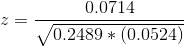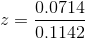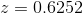P value :Is the value of the lowets level of singnificance at which we could reject the Null hypothesis H0

P value at 5% los

P value = 0.5318 > 0.5

Failed to Reject the null hypothesis and result is not significant

## Related Solutions

##### The ages? (in years) of a random sample of elementary school kids are shown. Determine the?...
The ages? (in years) of a random sample of elementary school kids are shown. Determine the? range, variance, and standard deviation of the sample data set. 5 7 9 6 7 8
##### A random sample of elementary school children in New York state is to be selected to...
A random sample of elementary school children in New York state is to be selected to estimate the proportion p p who have received a medical examination during the past year. It is desired that the sample proportion be within 0.045 of the true proportion with a 98 98 % level of confidence (a) Assuming no prior information about p p is available, approximately how large of a sample size is needed? If a planning study indicates that pp is...
##### A sample of 52 Elementary Statistics students includes 13 women. Assuming the sample is random: (a)...
A sample of 52 Elementary Statistics students includes 13 women. Assuming the sample is random: (a) Estimate the percentage of women taking Elementary Statistics by computing an appropriate confidence interval with 98% confidence. (b) At 10% significance, test whether less than 40% of the enrollment in all Elementary Statistics classes consists of women.
##### Salaries for teachers in a particular elementary school district are normally distributed with a mean of...
Salaries for teachers in a particular elementary school district are normally distributed with a mean of $44,000 and a standard deviation of$6,500. We randomly survey ten teachers from that district. 1.Find the probability that the teachers earn a total of over $400,000 2.If we surveyed 70 teachers instead of ten, graphically, how would that change the distribution in part d? 3.If each of the 70 teachers received a$3,000 raise, graphically, how would that change the distribution in part...
##### Salaries for teachers in a particular elementary school district are normally distributed with a mean of...
Salaries for teachers in a particular elementary school district are normally distributed with a mean of $44,000 and a standard deviation of$6,500. We randomly survey ten teachers from that district. Find the 85th percentile for the sum of the sampled teacher's salaries to 2 decimal places.